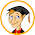## Wednesday, 11 January 2012

### Doubling and halving temperatures

I overheard a weather forecaster recently comparing the temperature one day with that forecast for the following day and telling us that temperatures tomorrow will be double what they are today!

Sorry, you can't do that! Of course, you can double the numbers, say, from 6 degrees C to 12 degrees C. But it is meaningless to do so. You cannot compare two temperatures in degrees C (or in degrees F) by considering their ratio. The implication is that in some sense one day is going to be 'twice as hot' as the previous day? That's nonsense!

There are different kinds of numerical scales and for each of these there are only certain kinds of numerical operations that are permissible and meaningful.

For example, you would not be doing anything meaningful by multiplying the number of a bus by three: three number 7 buses are not in any sense the same as a number 21 bus! Nor is a number 21 bus in some sense equivalent to three number 7s! Bus numbers are an example of a nominal scale, where the numbers are used as little more than names or labels for the buses. Using numbers allows us to list the buses in numerical order, and that's all. But there is no other context or situation where a number bus has to be followed by a number 8 bus.

If the numbers in the scale are used not just to name things but to recognise that they naturally come in order, then it is called an ordinal scale. For example, the scale used for assessing children in various school subjects – level 1, level 2, level 3, and so on – is an ordinal scale. Level 2 has to come in order between level 1 and level 3, because it is a higher level of achievement that level 1 and a lower level of achievement than level 3. But that's all you can do with an ordinal scale: put things (e.g. children) in order. There is no implication that the difference between achieving a level 2 and achieving a level 3 is in any sense the same as the difference between achieving a level 3 and achieving a level 4. So, you cannot do arithmetic with these numbers. For example, finding the mean average level in mathematics achieved by the children in a class by adding up the levels and dividing by the number of children would be unjustified, unacceptable and barmy!

In the forthcoming Olympics, the races in the athletics finals will put competitors in order: 1st, 2nd, 3rd, 4th, and so on (an ordinal scale), regardless of how big the gap might be from one to the next. The person who comes second in the 10,000 metres final could be 20 cm behind the leader or 200 metres, and still be 'number 2' across the finishing line.

But in some scales the numbers imply not just an order, but also a fixed interval from one to the next. A temperature scale is an example of this: it is called an interval scale. This means, for example, that the increase from 7 degrees to 8 degrees is in some sense the same as the increase from 8 degrees to 9 degrees, and from 9 degrees to 10 degrees, and so on. [Contrast this property with bus numbers: you can't talk about the increase from a number 7 bus to a number 8 bus as though it is some kind of measurable interval!]

In an interval scale, you can compare measurements only by looking at the difference between them, not by looking at their ratio. So, it's OK to say that the temperature forecast for tomorrow (12 degrees) will be 6 degrees higher than today (6 degrees). Yes, it will be 6 degrees hotter tomorrow than today! But it won't be twice as hot!

To see what I am getting at, ask the question, what would be twice as hot as 0 degrees? If you double 0 you get 0, of course. If 0 degrees is twice as hot as itself, then it would mean that there is a complete absence of heat on a day when the temperature is zero. [This might be true theoretically if we were using a scale where 0 degrees meant 'absolute zero'.] Although it might be chilly when it's 0 degrees C outside, we could not say that there is a complete absence of heat – because we know it can get colder than this! The temperature of 0 degrees C is not 'nothing'!

Only in scales where zero in some sense means 'nothing' can we use ratios to compare measurements. These are called, not surprisingly, ratio scales. Many familiar measurements are ratio scales: length, mass, capacity, and so on. For example, the capacity of one bottle is 250 ml and that of another is 750 ml. Now it is perfectly meaningful to say that the capacity of the larger bottle is 3 times that of the smaller bottle. Drinking 750 ml is the same as drinking 3 lots of 250 ml. And note the important condition for a ratio scale: a bottle with a capacity of zero millilitres (such as a solid wooden bottle that cannot hold any liquid) means there is nothing at all to drink.

#### 1 comment:

1.This is a nice clear explanation of data types, Derek. My students need to read this when writing reports. (Mind you, so do some academics in non-maths fields writing research papers.)